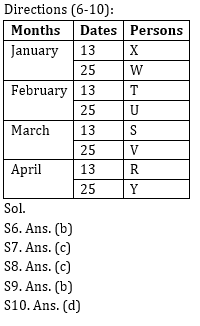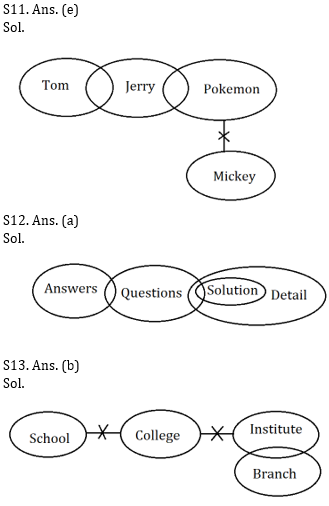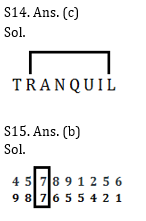# Reasoning Ability Quiz for Prelims Exams- SBI & IBPS 2020- 10th December

Directions (1-5): Study the following arrangement carefully and answer the questions given below:
3 1 2 5 4 7 2 8 4 5 7 1 9 6 5 2 4 1 4 5 8 2 4 3 9 7 6 9 4 2 3

Q1. Which of the following digit will be sixth to the left of tenth from the right end?
(a) 6
(b) 9
(c) 5
(d) 2
(e) None of these

Q2. How many 2’s is there in the given series which are immediately followed by a number less than 6?
(a) One
(b) None
(c) Three
(d) Two
(e) More than three

Q3. What will be the addition of fifth, seventh and tenth digit from the left end?
(a) 13
(b) 10
(c) 11
(d) 15
(e) None of these

Q4. If all the perfect square digits are removed from the given series (excluding 1), then which of the following will be fifth to the right of sixth from the left end?
(a) 5
(b) 2
(c) 8
(d) 1
(e) None of these

Q5. How many odd numbers are there in the given series which are immediately followed by a perfect square digit?
(a) One
(b) None
(c) Three
(d) Five
(e) Seven

Directions (6-10): Study the following information carefully and answer the questions given below:
Eight persons i.e., R, S, T, U, V, W, X and Y are working in an office. Each person takes one day leave in different days either on 13th or 25th of the four different months i.e., January, February, March and April but not necessarily in same order.
U takes leave before V but doesn’t take leave in the month of 31 days. There are three persons take leave between U and Y. R and S takes leave on prime numbered date. There are as many persons take leave before R as after W. Neither T nor X takes leave on 25th of the month. T takes leave before S and after X.

Q6. Who among the following takes leave on 13th April?
(a) X
(b) R
(c) T
(d) S
(e) None of these

Q7. How many persons take leave between Y and W?
(a) Three
(b) Two
(c) Five
(d) Four
(e) None of these

Q8. When V takes leave?
(a) 25th February
(b) 25th January
(c) 25th March
(d) 25th April
(e) None of these

Q9. Which among the following is true regarding X?
(a) No one takes leave after X
(b) Three persons take leave between X and S
(c) X takes leave on 25th January
(d) W takes leave before X
(e) None is true

Q10. Four of the following five are alike in certain way based from a group, find the one which does not belong to that group?
(a) S – 13th April
(b) V – 25th February
(c) U – 25th March
(d) W – 25th January
(e) U – 13th January

Directions (11-13): In each of the questions below are given some statements followed by some conclusions. You have to take the given statements to be true even if they seem to be at variance with commonly known facts. Read all the conclusions and then decide which of the given conclusions logically follows from the given statements disregarding commonly known facts.
(a) If only conclusion I follows.
(b) If only conclusion II follows.
(c) If either conclusion I or II follows
(d) If neither conclusion I nor II follows.
(e) If both conclusions I and II follow.

Q11. Statements: Some Tom is Jerry
Some Jerry is Pokemon
No Pokemon is Mickey
Conclusion: I. All Tom can be Pokemon
II. Some Mickey can be Jerry

Q12. Statements: Only a few Answers are Questions
Some Questions are Solutions
All Solutions are Details
Conclusions: I. All Questions being Answers is a possibility
II. Some Details can be Questions

Q13. Statements: No School is College
No College is Institute
Only a few Institute is Branch
Conclusions: I. All Institute can be Branch
II. Some Branch is not College

Q14. In the word ‘TRANQUIL’, how many pairs of the letters have the same number of letters between them in the word as in alphabet from the both forward and backward directions?
(a) Four
(b) Two
(c) One
(d) Three
(e) More than four

Q15. How many such numerals are there in the number ‘457891256’ which will remain at the same position when arranged in descending order from left to right?
(a) None
(b) One
(c) Two
(d) Three
(e) More than three

Practice More Questions of Reasoning for Competitive Exams:

##### Prelims Quiz Study Plan for SBI & IBPS Exams 2020

Solutions

S1. Ans(d)
Sol. The 16th element from the right end = ‘2’.

S2. Ans(e)
Sol. There are four 2’s which are followed by number less than 6 i.e., ‘2 5, 2 4, 2 4 and 2 3’.

S3. Ans(c)
Sol. The fifth, seventh and tenth digit from the left end are ‘4, 2 and 5′.
Hence, the sum is = (4+2+5) = 11.

S4. Ans(b)
Sol. After removing all the perfect square digits ‘3 2 5 7 2 8 5 7 6 5 2 5 8 2 3 7 6 2 3’
Then (6+5) = 11th digit from the left is ‘2’.

S5. Ans(e)
Sol. There are seven odd digits which are followed by a perfect square number- ‘3 1, 5 4, 7 1, 1 9, 1 4, 3 9, 9 4’’Practice with Crash Course and Online Test Series for IBPS Clerk Prelims: